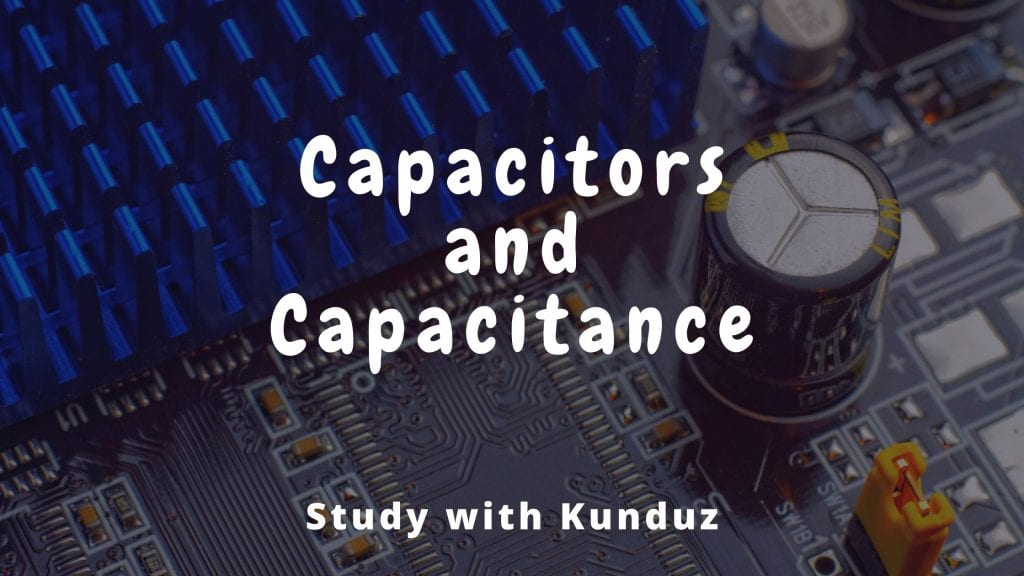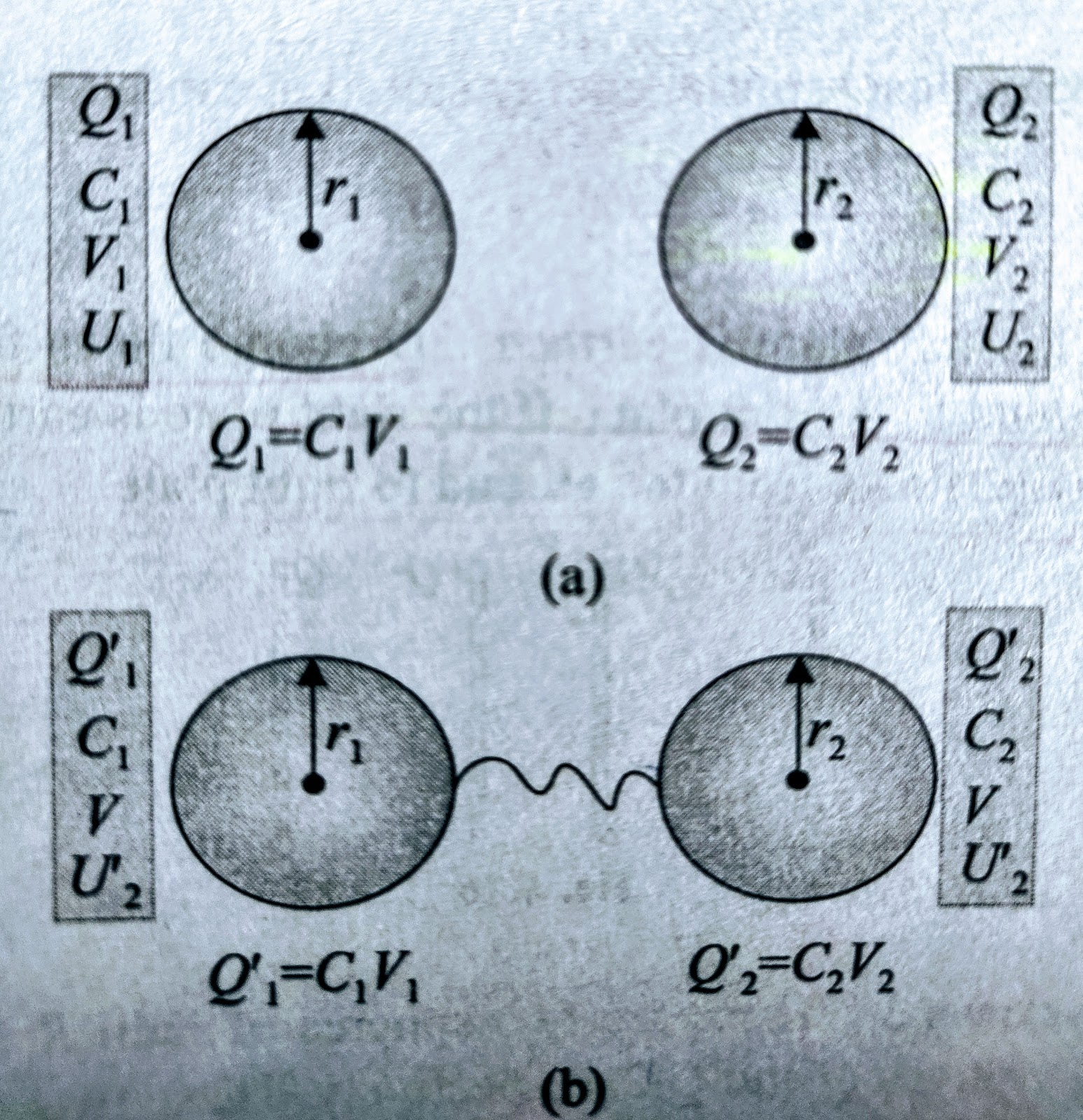Study Material

# Capacitor and Capacitance – Types, Formulae, Combination

This blog contains capacitors (parallel plate, spherical, cylindrical capacitors) and their capacitance formulae. Further, it covers series and parallel combination of capacitors. Also, it tells you what happens when two charged conductors are connected by conducting wire.

6 minutes long
Posted by Mahak Jain, 26/10/2020Hesap Oluştur

Got stuck on homework? Get your step-by-step solutions from real tutors in minutes! 24/7. Unlimited.

Capacitor is a device that stores electrostatic field energy. Capacitor provides temporary storage of energy in circuits and release the stored energy when required. Capacitance is the measure of energy that a capacitor can store.

## Capacitance

Potential of an isolated conductor is directly proportional to charge present on it .

V α Q

Inserting constant of proportionality

Q = C x V

or C = Q / V

Q / V is constant for isolated conductor and is defined as Capacitance of conductor .

If we assume, V = 1 volt

then  C = Q

Hence , Capacitance of an isolated conductor is charge required to rise the potential of isolated conductor by 1 volt .

#### Units of capacitance –

SI unit for capacitance is Farad .

## Spherical Capacitor and its Capacitance

Spherical capacitor consists of a solid or a hollow spherical conductor surrounded by another concentric hollow spherical conductor. The outer shell is earthed .

Suppose a charge +Q is present on the inner shell. Due to +Q charge on inner shell, there is charge – Q (induced) on the inner surface of the outer shell and + Q on the outer surface of the outer shell (as shown in following figure). Since, outer shell is earthed that’s why charge +Q will flow from the outer shell to the Earth.

Capacitance ( C ) = Q / potential difference

As we know outer shell is earthed that’s why potential on outer shell is zero.

Potential on inner shell = ( Q/4πε₀ ) [1/b – 1/a]

⇒ C = Q/ V₂= 4πε₀ab/(b-a)

## Cylindrical Capacitor and its Capacitance

Cylindrical capacitor consist of a solid or hollow cylindrical conductor surrounded by another coaxial hollow cylindrical conductor .

Potential difference = (q / 2πε₀L) ln(r₂/r₁)

Capacitance = 2πε₀L/ ln (r₂/r₁)

## Parallel Plate Capacitor and its Capacitance

A parallel plate capacitor consists of two large plates placed parallel to each other with a separation d. d is very small in comparison to the length and breadth of plates.

We know that electric field near a very large sheet is given by σ /2ε₀

Thus, Electric field between the plates is,

E = σ/2ε₀ + σ/2ε₀ = σ/ε₀ = Q/Aε₀ {σ = Q/A}

Potential difference between the plates is,

V = Ed = Qd/Aε₀

⇒ C = Q /V = ε₀A / d

### Factors affecting Capacitance –

Capacitance is function of physical dimensions of the conductor and permittivity of the dielectric present inside it. So, Capacitance mainly depends upon area of plate , distance between plate and dielectric present inside it

•   Area –

Capacitance of parallel plate capacitor is proportional to area of plates. All other factors being equal , greater plat area gives grater capacitance and less plate area gives less capacitance.

•   Distance between plates-

Capacitance of parallel plate capacitor is inversely proportional to distance between plates. All the factors being equal further plate spacing gives less capacitance, closer plate spacing gives more capacitance.

• Capacity of Parallel Plate capacitor with dielectric –

Suppose a parallel plate capacitor with area A and a separation d. A dielectric slab of thickness t, area A and dielectric constant K is inserted between the plates.

If Q charge is given to the capacitor plates then,

Electric field between the plates of the capacitor is outside the slab –

E₀ = σ / ε₀ = Q / Aε₀

Electric field inside dielectric slab –

E= E₀/ K = Q / Kε₀A

Potential difference between plate –

V = V₁ + V₂ + V₃

⇒  Q / C = E₀ t₁ + E t₂ + E₀ t₃

C = [ε₀ A / (d-t)] + t/k

##### Special Cases :
• for conducting slab (metal plate) –

Dielectric constant (K) = ∞ (infinity)

⇒ t / K = 0

⇒ C = ε₀A /(d-t)

• for conducting slab with t <<< d –

C = ε₀ A / d

## Series Combination of Capacitors

Suppose three capacitors with capacitance C₁, C₂ and C₃ are connected in series with a battery of voltage V as shown in figure.

In series combination V = V₁ + V₂ + V₃

⇒ Q / C = Q / C₁ + Q/ C₂ + Q/ C₃

Thus, 1/ C = 1/C₁ + 1/C₂ + 1/C₃

• In series combination equivalent capacitance is always less than any of the individual capacitance.
• Charge on capacitors are same but potential across the capacitors are different.

## Parallel Combination of Capacitors

Suppose three capacitor with capacitance C₁, C₂ and C₃ are connected in parallel with a battery of voltage V as shown in fig.

In parallel combination q = q₁ + q₂ + q₃

⇒ CV = C₁V + C₂V + C₃V

Thus, C = C₁ + C₂ + C₃

• In parallel combination equivalent capacitance is always greater than any of the individual capacitance.
• Charge on capacitors are different but potential across the capacitors are same.

## Sharing of Charges

When two conductors are connected by a connecting wire, charge flows from one conductor to another (higher potential to lower potential). This charge flow phenomenon takes place until both the conductor have same potential.Q₁+Q₂=Q₁´+Q₂´ =Q

Q₂´= Q[r₂/ (r₂+ r₁)]

Q₁´= Q[r₁/ (r₁+r₂)]

Common potential,V = C₁V₁+C₂V₂/(C₁+C₂)

Therefore, Energy loss =[ C₁C₂/2(C₁+C₂)] (V₁-V₂)²